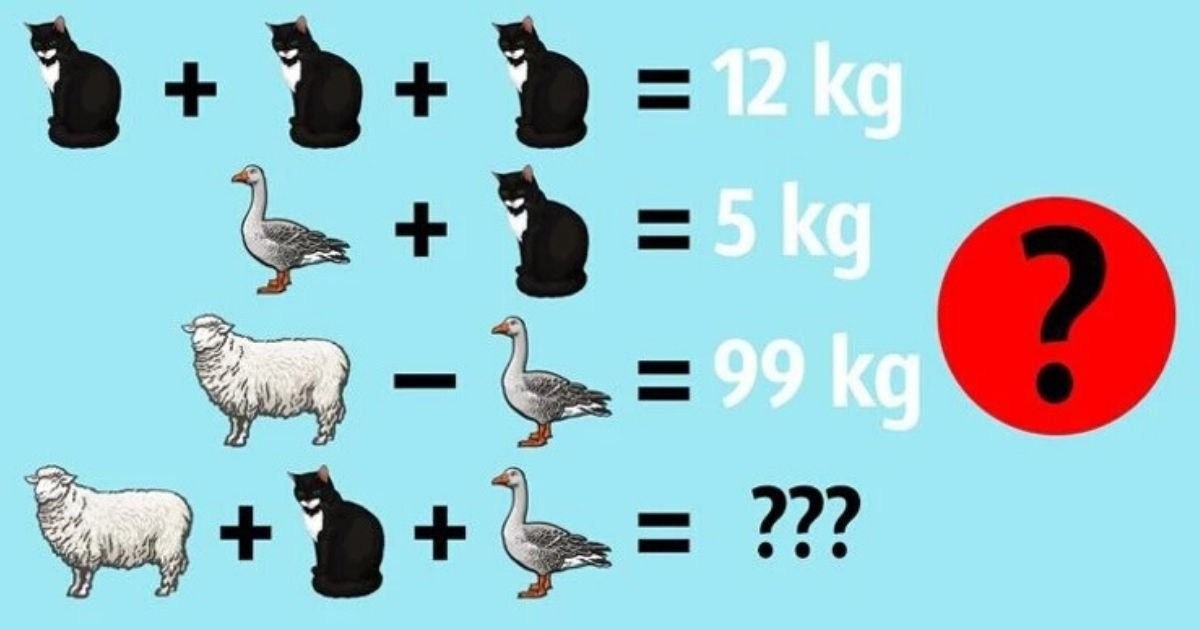# Can You Solve This Math Puzzle For Kids? Most Adults Struggle To Answer Correctly!

Following its appearance on social media, a simple yet complex mathematical conundrum has been driving people insane.

You’ll have to conduct some basic math while keeping an eye on your answers and the symbols you come across in this assignment.As you can see, your task is to calculate the combined weight of the sheep, cat, and duck. You’ll need to rely on the information supplied in the first three lines to arrive at the correct answer.

Check out the solution below once you’re confident you’ve got the right answer!

The solution to this arithmetic problem is 105, which means that all three animals collectively weigh 105 kilos. See the breakdown of the problem below if you’re not clear how we arrived at that figure.

Let’s start with the assumption that C stands for cat, D for duck, and S for sheep.

LINE 1: C + C + C = 12, meaning that 3C = 12 and C = 4. Therefore, the weight of the cat is 4 kilograms.

LINE 2: D + C = 5, therefore D + 4 = 5, meaning that D = 1. The weight of the duck is 1 kilogram.

LINE 3: S – D = 99, therefore S – 1 = 99, meaning that S = 100. The weight of the sheep is 100 kilograms.

Once you’re done with the first three lines, simply add the three weights together to get the correct answer.

LINE 4: S + C + D = 100 + 4 + 1 = 105. The answer is 105!

Were you able to answer properly without our assistance? If so, please tell us how long it took you. If not, feel free to try again with the math problem!